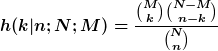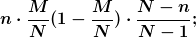# Why is the hypergeometric distribution important

## hypergeometric distribution

special discrete probability distribution with the probability functionfor k with M + n - N ≤ k ≤ min (n, M), where n, M and N-M are natural numbers with N-M ≤ n ≤ N. The expressions used in the probability function are binomial coefficients. The hypergeometric distribution covers the following fact: In a population of size N there are two types of elements. The number of elements of the first type is M, that of the second types N - M. N elements are randomly removed without replacing (urn model). Then h (k | n; N; M) gives the probability that exactly k elements of the first kind will be included in the drawing. The hypergeometric distribution has the parameters n, M and N. The hypergeometric distribution is used, for example, in the so-called good / bad test in the context of quality control through a sample of goods. The expected value of a hypergeometrically distributed random variable is n · M / N and the variance(N - n) / (N - 1) is the so-called correction factor. Under certain conditions, the hypergeometric distribution can be approximated by the binomial distribution and the normal distribution (approximation).